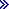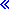# %LOOKUPxx (Look Up an Array Element)``````%LOOKUP(arg : array | keyed_array_DS {: start_index {: number_of_elements}})
%LOOKUPLT(arg : array  {: start_index {: number_of_elements}})
%LOOKUPGE(arg : array  {: start_index {: number_of_elements}})
%LOOKUPGT(arg : array  {: start_index {: number_of_elements}})
%LOOKUPLE(arg : array  {: start_index {: number_of_elements}})``````The following functions return the array index of the item in the array that matches that matches arg. %LOOKUP can also be used to return the array index of the item in a keyed array data structure.
%LOOKUP
An exact match.
%LOOKUPLT
The value that is closest to arg but less than arg.
%LOOKUPLE
An exact match, or the value that is closest to arg but less than arg.
%LOOKUPGT
The value that is closest to arg but greater than arg.
%LOOKUPGE
An exact match, or the value that is closest to arg but greater than arg.If no value matches the specified condition, zero is returned. The value returned is in unsigned integer format (type U).There could be more than one element that matches the specified condition. For the following discussion, assume the following values for the ascending and descending arrays.
``````           +---+---+---+---+---+---+---+
| 1 | 2 | 3 | 4 | 5 | 6 | 7 |
-----------+---+---+---+---+---+---+---+
ASCEND     | A | C | C | C | E | E | G |
-----------+---+---+---+---+---+---+---+
DESCEND    | G | E | E | E | C | C | A |
-----------+---+---+---+---+---+---+---+``````
• For %LOOKUP, %LOOKUPLE, or %LOOKUPGE, if there is more than one element that is equal to the search argument, then the index of the first matching element is returned. For example, if the search argument is C, these built-in functions will return 2 for the ascending array and 5 for the descending array.
• For %LOOKUPLE for ascending arrays, if there is no element that is equal to the search argument, then the built-in function returns the index of the last element less than the search argument. For example, if the search argument is D, %LOOKUPLE returns 4.
• For %LOOKUPLE for descending arrays, if there is no element that is equal to the search argument, then the built-in function returns the index of the first element less than the search argument. For example, if the search argument is D, %LOOKUPLE returns 5.
• For %LOOKUPGE for ascending arrays, if there is no element that is equal to the search argument, then the built-in function returns the index of the first element greater than the search argument. For example, if the search argument is D, %LOOKUPGE returns 5.
• For %LOOKUPGE for descending arrays, if there is no element that is equal to the search argument, then the built-in function returns the index of the last element greater than the search argument. For example, if the search argument is D, %LOOKUPGE returns 4.
• For %LOOKUPLT for ascending arrays, the built-in function returns the index of the last element less than the search argument. For example, if the search argument is D, %LOOKUPLT returns 4.
• For %LOOKUPLT for descending arrays, the built-in function returns the index of the first element less than the search argument. For example, if the search argument is D, %LOOKUPLT returns 5.
• For %LOOKUPGT for ascending arrays, the built-in function returns the index of the first element greater than the search argument. For example, if the search argument is D, %LOOKUPGT returns 5.
• For %LOOKUPGT for descending arrays, the built-in function returns the index of the last element greater than the search argument. For example, if the search argument is D, %LOOKUPGT returns 4.The search starts at index start_index and continues for number_of_elems elements. By default, the entire array is searched.The second parameter can be a scalar array in the form ARRAY_NAME. For %LOOKUP, it can also be a keyed array data structure in the form ARRAY_DS_NAME(*).SUBFIELD_NAME.To search an array data structure, specify the data structure name with an index of (*), then specify the subfield to be used as the key for the search. For example, to search for a value of 'XP2' in the CODE subfield of array data structure INFO, specify 'XP2' as the first parameter and specify INFO(*).CODE as the second parameter. The part of the qualified name up to the (*) index must represent an array, and the part of the qualified name after the (*) must represent a scalar subfield, or indexed array of scalars.

The first two parameters can have any type but must have the same type. For a keyed data structure array, the first parameter must have the same type as the key. They do not need to have the same length or number of decimal positions. The third and fourth parameters must be non-float numeric values with zero decimal positions.

For %LOOKUPLT, %LOOKUPLE, %LOOKUPGT, and %LOOKUPGE, the array must be defined with keyword ASCEND or DESCEND. The ALTSEQ table is used, unless arg or array is defined with ALTSEQ(*NONE).

Built-in functions %FOUND and %EQUAL are not set following a %LOOKUP operation.

The %LOOKUPxx built-in functions use a binary search for sequenced arrays (arrays that have the ASCEND or DESCEND keyword specified).

Note: Unlike the LOOKUP operation code, %LOOKUP applies only to arrays. To look up a value in a table, use the %TLOOKUP built-in function.
Figure 1. %LOOKUPxx with a scalar array
`````` *..1....+....2....+....3....+....4....+....5....+....6....+....7...+....
/FREE
arr(1) = 'Cornwall';
arr(2) = 'Kingston';
arr(3) = 'London';
arr(4) = 'Paris';
arr(5) = 'Scarborough';
arr(6) = 'York';

n = %LOOKUP('Paris':arr);
// n = 4

n = %LOOKUP('Thunder Bay':arr);

n = %LOOKUP('Kingston':arr:3);

n = %LOOKUPLE('Paris':arr);
// n = 4

n = %LOOKUPLE('Milton':arr);
// n = 3

n = %LOOKUPGT('Sudbury':arr);
// n = 6

n = %LOOKUPGT('Yorks':arr:2:4);
/END-FREE``````
Figure 2. %LOOKUP with an array data structure
``````D emps            DS                  QUALIFIED DIM(20)
D    name                       25A   VARYING
D    id                          9S 0
D numEmps         S             10I 0
/FREE
emps(1).name = 'Mary';
emps(1).id = 00138;
emps(2).name = 'Patrick';
emps(2).id = 10379;
emps(3).name = 'Juan';
emps(3).id = 06254;
numEmps = 3;

// Search for employee 'Patrick'
n = %lookup('Patrick' : emps(*).name : 1 : numEmps);
// n = 2

// Search for the employee with id 06254
n = %lookup(06254 : emps(*).id : 1 : numEmps);
// n = 3